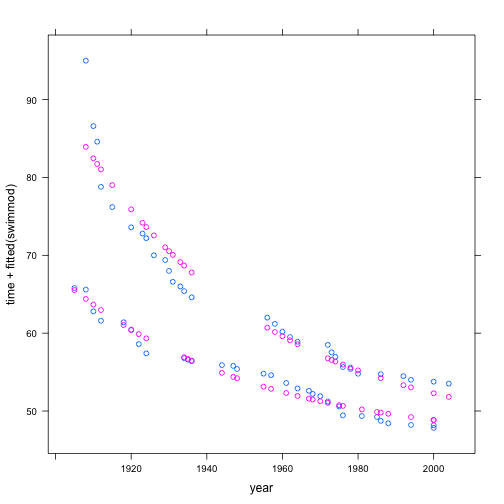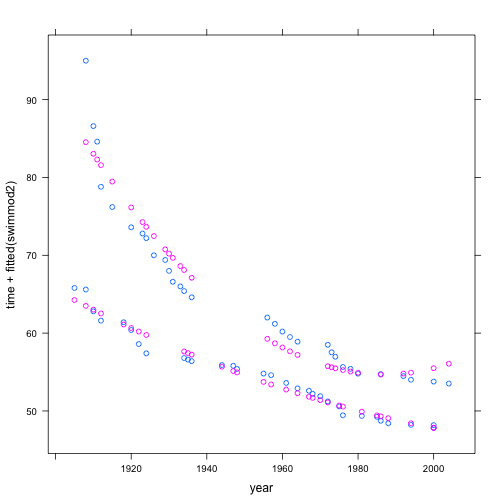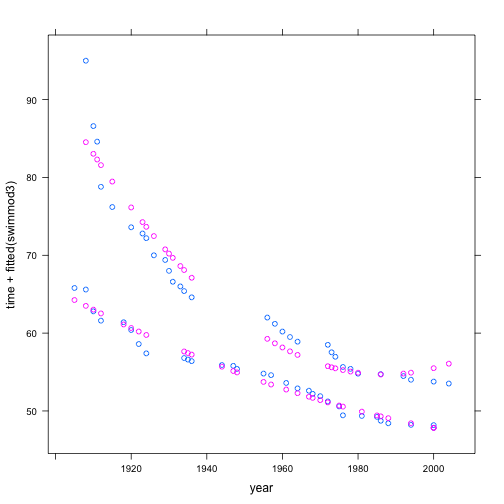# February 18, 2013 Class Notes

### Back to RMarkdown

Several people had problems compiling their documents. These were caused by ill-formed commands earlier in the document.

Use the “Chunks” menu to run individual chunks by copying them to your console.

Write up a short document about partitioning variance and post it to Moodle.

### Notation

In the lm() program (and mm() and glm() programs), we write the response variable to the left of the ~ and the model terms, separated by the + notation, on the right side. Don't confuse + with mathematical addition. It really is just a separator for the various terms we are going to be including in the linear combination that will constitute the model.

• The 1 means “intercept”. As it happens, the software will automatically include the intercept even if you don't say 1. If you want to exclude the intercept, you use a special notation: -1.

Example of simple models with and without the intercept term.

lm(height ~ 1 + father, data = Galton)

##
## Call:
## lm(formula = height ~ 1 + father, data = Galton)
##
## Coefficients:
## (Intercept)       father
##      39.110        0.399

lm(height ~ father, data = Galton)  # same thing as previous

##
## Call:
## lm(formula = height ~ father, data = Galton)
##
## Coefficients:
## (Intercept)       father
##      39.110        0.399

lm(height ~ father - 1, data = Galton)  # leave out intercept

##
## Call:
## lm(formula = height ~ father - 1, data = Galton)
##
## Coefficients:
## father
##  0.964

• The name of the variable, e.g. father means that variable as a main effect.

• An interaction term is introduced with a : between variable names.

lm(height ~ father + mother + father:mother, data = Galton)

##
## Call:
## lm(formula = height ~ father + mother + father:mother, data = Galton)
##
## Coefficients:
##   (Intercept)         father         mother  father:mother
##      132.3478        -1.2060        -1.4294         0.0247

• Since the polynomial modeling heuristic includes main effects by default, there is a special notation to signal that we want both the main effects and the interaction:
lm(height ~ father * mother, data = Galton)

##
## Call:
## lm(formula = height ~ father * mother, data = Galton)
##
## Coefficients:
##   (Intercept)         father         mother  father:mother
##      132.3478        -1.2060        -1.4294         0.0247

• Transformation terms: apply the function to the variable. Example:
lm(height ~ cos(father), data = Galton)  # silly model!

##
## Call:
## lm(formula = height ~ cos(father), data = Galton)
##
## Coefficients:
## (Intercept)  cos(father)
##     66.7618      -0.0094

swim = fetchData("swim100m.csv")

## Retrieving from http://www.mosaic-web.org/go/datasets/swim100m.csv

swimmod = lm(time ~ sex * exp(-year/50), data = swim)
xyplot(time + fitted(swimmod) ~ year, data = swim)(N.B.: For instructors … Beware that the above exponential model just barely makes it within the floating-point arithmetic capabilities of the computer. Applying an exponential to numbers arguments like $$-20$$ can be perilous. Better to have rescaled year to, say, start at 1900.)

• Quadratic terms are fairly common, and so there is a nice way to specify that you want both the main effect and higher-order polynomial terms:
swimmod2 = lm(time ~ sex * poly(year, 2), data = swim)
xyplot(time + fitted(swimmod2) ~ year, data = swim)N.B. For instructors … You may be tempted to write polynomial terms directly, for instance

swimmod3 = lm(time ~ sex * (year + I(year^2)), data = swim)
xyplot(time + fitted(swimmod3) ~ year, data = swim)Note the use of I() to instruct R to treat arithmetical symbols in the numerical way. Also, note that poly() cleverly does a shift into orthogonal polynomials to preserve numerical stability. As such, the specific coefficients in swimmod2 and swimmod3 will be different, even though the model formulas amount to the same thing.

swimmod2

##
## Call:
## lm(formula = time ~ sex * poly(year, 2), data = swim)
##
## Coefficients:
##         (Intercept)                 sexM       poly(year, 2)1
##               64.57                -9.64               -76.13
##      poly(year, 2)2  sexM:poly(year, 2)1  sexM:poly(year, 2)2
##               27.23                36.31               -22.49

swimmod3

##
## Call:
## lm(formula = time ~ sex * (year + I(year^2)), data = swim)
##
## Coefficients:
##    (Intercept)            sexM            year       I(year^2)
##       1.90e+04       -1.54e+04       -1.90e+01        4.79e-03
##      sexM:year  sexM:I(year^2)
##       1.56e+01       -3.96e-03


In order to deal with the possible double meaning of symbols like +, you can use I() to signal to R to treat the mathematical operations literally.

## Introducing $$R^2$$ Briefly

Since we're trying to account for variation in the response variable, it's helpful to have a way to quantify how much variation we have accounted for. The quantity called “R-squared” — $$R^2$$, officially “the coefficient of determination” although hardly anyone uses that name — is a standard way to do this. Briefly, $$R^2$$ is the fraction of variance in the response variable that is “captured” by the model. It is always between 0 (didn't capture any) and 1 (got it all!).

### Activity

Visual choice of model terms

Instructions are given here (These have to be reformatted as a handout and as an instructor's guide.)

The only substantive differences is in the way the software is loaded:

fetchData("mLM.R")

## Retrieving from http://www.mosaic-web.org/go/datasets/mLM.R

##  TRUE


The program itself is called mLM() (not lm.select.terms())

## Model Formulas and Coefficients

Fit some models and work students through the calculations. The makeFun() function can help in this.

A suggested style for a calculation:

mod = lm(wage ~ sex + age, data = CPS85)
coef(mod)

## (Intercept)        sexM         age
##     4.65425     2.27469     0.08522


What is the wage for a female of age 40? A male of age 30? Work through the arithmetic “by hand”.

4.65 + 0 * 2.274 + 40 * 0.0852  # for the 40-year old female

##  8.058

4.65 + 1 * 2.274 + 30 * 0.0852  # for the 30-year old male

##  9.48


You can also turn the model information into a mathematical function:

f = makeFun(mod)
f(sex = "F", age = 40)

##     1
## 8.063

f(sex = "M", age = 30)

##     1
## 9.485


Other settings:

• Natural gas usage by average temperature in utilities.csv
• … more …

## Form Groups for Car Project

Outline the used car project and show some data from http://www.cars.com. Perhaps MINI Coopers.

Get students to form groups right now, oriented around different kinds of cars. They should make a Google Spreadsheet and share it with all the other members of their group.